Case Based Questions Test: Polynomials - 1

# Case Based Questions Test: Polynomials - 1 - Class 10

Test Description

## 15 Questions MCQ Test Mathematics (Maths) Class 10 - Case Based Questions Test: Polynomials - 1

Case Based Questions Test: Polynomials - 1 for Class 10 2023 is part of Mathematics (Maths) Class 10 preparation. The Case Based Questions Test: Polynomials - 1 questions and answers have been prepared according to the Class 10 exam syllabus.The Case Based Questions Test: Polynomials - 1 MCQs are made for Class 10 2023 Exam. Find important definitions, questions, notes, meanings, examples, exercises, MCQs and online tests for Case Based Questions Test: Polynomials - 1 below.
Solutions of Case Based Questions Test: Polynomials - 1 questions in English are available as part of our Mathematics (Maths) Class 10 for Class 10 & Case Based Questions Test: Polynomials - 1 solutions in Hindi for Mathematics (Maths) Class 10 course. Download more important topics, notes, lectures and mock test series for Class 10 Exam by signing up for free. Attempt Case Based Questions Test: Polynomials - 1 | 15 questions in 30 minutes | Mock test for Class 10 preparation | Free important questions MCQ to study Mathematics (Maths) Class 10 for Class 10 Exam | Download free PDF with solutions
 1 Crore+ students have signed up on EduRev. Have you?
Case Based Questions Test: Polynomials - 1 - Question 1

### Direction: Read the following text and answer the following questions on the basis of the same:The below picture are few natural examples of parabolic shape which is represented by a quadratic polynomial. A parabolic arch is an arch in the shape of a parabola. In structures, their curve represents an efficient method of load, and so can be found in bridges and in architecture in a variety of forms.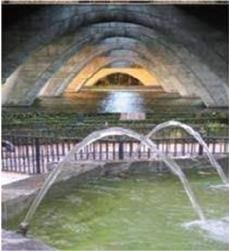If the sum of the roots is –p and product of the roots is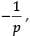then the quadratic polynomial is

Detailed Solution for Case Based Questions Test: Polynomials - 1 - Question 1

x2 - (Sum of roots)x + Product of roots

Putting values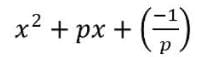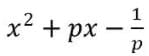We can multiply any constant to this polynomial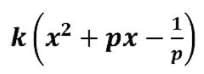Case Based Questions Test: Polynomials - 1 - Question 2

### Direction: Read the following text and answer the following questions on the basis of the same:The below picture are few natural examples of parabolic shape which is represented by a quadratic polynomial. A parabolic arch is an arch in the shape of a parabola. In structures, their curve represents an efficient method of load, and so can be found in bridges and in architecture in a variety of forms.If the roots of the quadratic polynomial are equal, where the discriminant D = b2 – 4ac, then

Detailed Solution for Case Based Questions Test: Polynomials - 1 - Question 2
If the roots of the quadratic polynomial are equal, then discriminant is equal to zero D = b2 – 4ac = 0
Case Based Questions Test: Polynomials - 1 - Question 3

### Direction: Read the following text and answer the following questions on the basis of the same:The below picture are few natural examples of parabolic shape which is represented by a quadratic polynomial. A parabolic arch is an arch in the shape of a parabola. In structures, their curve represents an efficient method of load, and so can be found in bridges and in architecture in a variety of forms.The graph of x2 + 1 = 0

Detailed Solution for Case Based Questions Test: Polynomials - 1 - Question 3
Graph each side of the equation.

y = x2 + 1

y = 0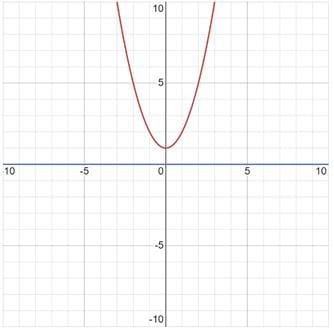Case Based Questions Test: Polynomials - 1 - Question 4

Direction: Read the following text and answer the following questions on the basis of the same:
The below picture are few natural examples of parabolic shape which is represented by a quadratic polynomial. A parabolic arch is an arch in the shape of a parabola. In structures, their curve represents an efficient method of load, and so can be found in bridges and in architecture in a variety of forms.In the standard form of quadratic polynomial, ax2 + bx, c, a, b and c are

Detailed Solution for Case Based Questions Test: Polynomials - 1 - Question 4

It can be written in the standard form ax2 + bx + c , where x is a variable, a, b, c are constants (numbers) and a = 0. The constants a, b, c are called the coefficients of the polynomial. A quadratic polynomial ax2 + bx + c is called sometimes a quadratic trinomial.

Case Based Questions Test: Polynomials - 1 - Question 5

Direction: Read the following text and answer the following questions on the basis of the same:

The below picture are few natural examples of parabolic shape which is represented by a quadratic polynomial. A parabolic arch is an arch in the shape of a parabola. In structures, their curve represents an efficient method of load, and so can be found in bridges and in architecture in a variety of forms.If α are 1/α the zeroes of the quadratic polynomial 2x2 – x + 8k, then k is

Detailed Solution for Case Based Questions Test: Polynomials - 1 - Question 5
Given equation, 2x2 – x + 8k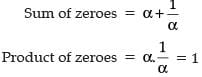Product of zeroes = c/a = 8k/2

So, 8k/2 = 1

k = 2/8

k = 1/4

Case Based Questions Test: Polynomials - 1 - Question 6

Direction: Read the following text and answer the following questions on the basis of the same:

Basketball and soccer are played with a spherical ball. Even though an athlete dribbles the ball in both sports, a basketball player uses his hands and a soccer player uses his feet. Usually, soccer is played outdoors on a large field and basketball is played indoor on a court made out of wood. The projectile (path traced) of soccer ball and basketball are in the form of parabola representing quadratic polynomial.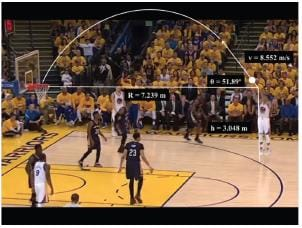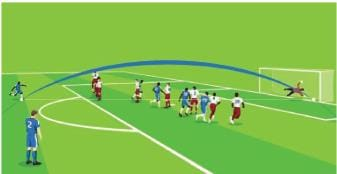What will be the expression of the polynomial?

Detailed Solution for Case Based Questions Test: Polynomials - 1 - Question 6
Since, the three zeroes = – 3, – 1, 2

Hence, the expression is (x + 3)(x + 1)(x – 2)

= [x2 + x + 3x + 3](x – 2)

= x3 + 4x2 + 3x – 2x2 – 8x – 6

= x3 + 2x2 – 5x – 6

Case Based Questions Test: Polynomials - 1 - Question 7

Direction: Read the following text and answer the following questions on the basis of the same:

Basketball and soccer are played with a spherical ball. Even though an athlete dribbles the ball in both sports, a basketball player uses his hands and a soccer player uses his feet. Usually, soccer is played outdoors on a large field and basketball is played indoor on a court made out of wood. The projectile (path traced) of soccer ball and basketball are in the form of parabola representing quadratic polynomial.The graph of parabola opens upwards, if _______

Detailed Solution for Case Based Questions Test: Polynomials - 1 - Question 7
The graph of y = ax2 + bx + c opens upwards when the coefficient of the highest degree term (i.e. x2) is greater than zero.

⇒ a > 0.

Case Based Questions Test: Polynomials - 1 - Question 8

Direction: Read the following text and answer the following questions on the basis of the same:

Basketball and soccer are played with a spherical ball. Even though an athlete dribbles the ball in both sports, a basketball player uses his hands and a soccer player uses his feet. Usually, soccer is played outdoors on a large field and basketball is played indoor on a court made out of wood. The projectile (path traced) of soccer ball and basketball are in the form of parabola representing quadratic polynomial.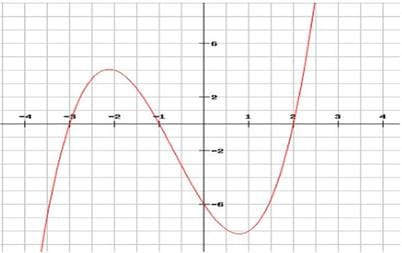The three zeroes in the above shown graph are

Detailed Solution for Case Based Questions Test: Polynomials - 1 - Question 8Here,

Zeroes are the values of x where graph intersects the x-axis

∴ Zeroes are -3, -1 and 2

Case Based Questions Test: Polynomials - 1 - Question 9

Direction: Read the following text and answer the following questions on the basis of the same:

Basketball and soccer are played with a spherical ball. Even though an athlete dribbles the ball in both sports, a basketball player uses his hands and a soccer player uses his feet. Usually, soccer is played outdoors on a large field and basketball is played indoor on a court made out of wood. The projectile (path traced) of soccer ball and basketball are in the form of parabola representing quadratic polynomial.The shape of the path traced shown is

Detailed Solution for Case Based Questions Test: Polynomials - 1 - Question 9
Parabola: In projectile motion, it is the shape of the path traced by a projectile.
Case Based Questions Test: Polynomials - 1 - Question 10

Direction: Read the following text and answer the following questions on the basis of the same:
Basketball and soccer are played with a spherical ball. Even though an athlete dribbles the ball in both sports, a basketball player uses his hands and a soccer player uses his feet. Usually, soccer is played outdoors on a large field and basketball is played indoor on a court made out of wood. The projectile (path traced) of soccer ball and basketball are in the form of parabola representing quadratic polynomial.Observe the following graph and answer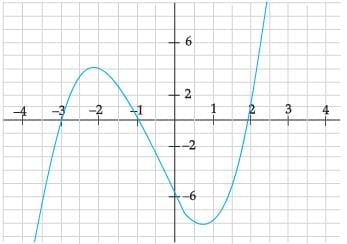In the above graph, how many zeroes are there for the polynomial?

Detailed Solution for Case Based Questions Test: Polynomials - 1 - Question 10

The number of zeroes of polynomial is the number of times the curve intersects the x-axis, i.e. attains the value 0.
Here, the polynomial meets the x-axis at 3 points.
So, number of zeroes = 3.

Case Based Questions Test: Polynomials - 1 - Question 11

Direction: Read the following text and answer the following questions on the basis of the same:

For a linear polynomial kx + c, k ≠ 0, the graph of y = kx + c is a straight line which intersects the X-axis at exactly one point, namely,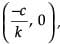Therefore, the linear polynomial kx + c, k ≠ 0, has exactly one zero, namely, the X-coordinate of the point where the graph of y = kx + c intersects the X-axis.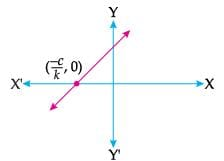If the product of the zeroes of the quadratic polynomial p(x) = ax2 – 6x – 6 is 4, then the value of a is:

Detailed Solution for Case Based Questions Test: Polynomials - 1 - Question 11
We have,

p(x) = ax2 – 6x – 6

Let α and β be the zeroes of the given polynomial, then

αβ = c/a

i.e., 4 = -6/a

⇒ 4a = – 6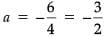Case Based Questions Test: Polynomials - 1 - Question 12

Direction: Read the following text and answer the following questions on the basis of the same:
For a linear polynomial kx + c, k ≠ 0, the graph of y = kx + c is a straight line which intersects the X-axis at exactly one point, namely,Therefore, the linear polynomial kx + c, k ≠ 0, has exactly one zero, namely, the X-coordinate of the point where the graph of y = kx + c intersects the X-axis.The graph of y = p(x) is given in figure below for some polynomial p(x). The number of zero/zeroes of p(x) is/are: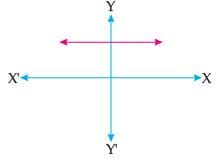Detailed Solution for Case Based Questions Test: Polynomials - 1 - Question 12

Since the graph does not intersect the X-axis, therefore it has no zero.

Case Based Questions Test: Polynomials - 1 - Question 13

Direction: Read the following text and answer the following questions on the basis of the same:

For a linear polynomial kx + c, k ≠ 0, the graph of y = kx + c is a straight line which intersects the X-axis at exactly one point, namely,Therefore, the linear polynomial kx + c, k ≠ 0, has exactly one zero, namely, the X-coordinate of the point where the graph of y = kx + c intersects the X-axis.If a and b are the zeroes of the quadratic polynomial p(x) = 4x2 + 5x + 1, then the product of zeroes is:

Detailed Solution for Case Based Questions Test: Polynomials - 1 - Question 13
We have, p(x) = 4x2 + 5x + 1

∴ αβ = c/a = 1/4.

Case Based Questions Test: Polynomials - 1 - Question 14

Direction: Read the following text and answer the following questions on the basis of the same:

For a linear polynomial kx + c, k ≠ 0, the graph of y = kx + c is a straight line which intersects the X-axis at exactly one point, namely,Therefore, the linear polynomial kx + c, k ≠ 0, has exactly one zero, namely, the X-coordinate of the point where the graph of y = kx + c intersects the X-axis.If a linear polynomial is 2x + 3, then the zero of 2x + 3 is:

Detailed Solution for Case Based Questions Test: Polynomials - 1 - Question 14
Given, polynomial = 2x + 3

Let p(x) = 2x + 3

For a zero of p(x), 2x + 3 = 0

⇒ 2x = – 3

⇒ x =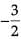Case Based Questions Test: Polynomials - 1 - Question 15

Direction: Read the following text and answer the following questions on the basis of the same:

For a linear polynomial kx + c, k ≠ 0, the graph of y = kx + c is a straight line which intersects the X-axis at exactly one point, namely,Therefore, the linear polynomial kx + c, k ≠ 0, has exactly one zero, namely, the X-coordinate of the point where the graph of y = kx + c intersects the X-axis.If α and β are the zeroes of the quadratic polynomial x2 – 5x + k such that α – β = 1, then the value of k is:

Detailed Solution for Case Based Questions Test: Polynomials - 1 - Question 15
∵ p(x) = x2 – 5x + k

Then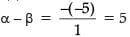and αβ = k/1 = k

Also given, α – β = 1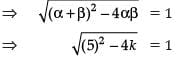⇒ 25 – 4k = 1(Squaring both sides)

⇒ – 4k = 1 – 25 = – 24

⇒ k = 6.

## Mathematics (Maths) Class 10

115 videos|478 docs|129 tests
Information about Case Based Questions Test: Polynomials - 1 Page
In this test you can find the Exam questions for Case Based Questions Test: Polynomials - 1 solved & explained in the simplest way possible. Besides giving Questions and answers for Case Based Questions Test: Polynomials - 1, EduRev gives you an ample number of Online tests for practice

## Mathematics (Maths) Class 10

115 videos|478 docs|129 tests